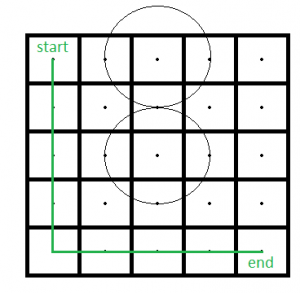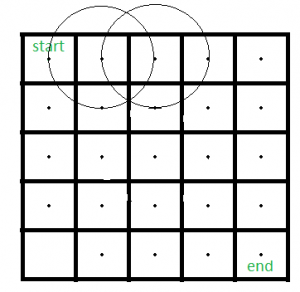# Path in a Rectangle with Circles

There is a m*n rectangular matrix whose top-left(start) location is (1, 1) and bottom-right(end) location is (m*n). There are k circles each with radius r. Find if there is any path from start to end without touching any circle.
The input contains values of m, n, k, r and two array of integers X and Y, each of length k. (X[i], Y[i]) is the center of ith circle.
Source : Directi Interview

Examples:

Attention reader! Don’t stop learning now. Get hold of all the important DSA concepts with the DSA Self Paced Course at a student-friendly price and become industry ready.  To complete your preparation from learning a language to DS Algo and many more,  please refer Complete Interview Preparation Course.

In case you wish to attend live classes with experts, please refer DSA Live Classes for Working Professionals and Competitive Programming Live for Students.

```Input : m = 5, n = 5, k = 2, r = 1,
X = {1, 3}, Y = {3, 3}
Output : Possible
```Here is a path from start to end point.

```Input : m = 5, n = 5, k = 2, r = 1,
X = {1, 1}, Y = {2, 3}.
Output : Not Possible
```Approach : Check if the centre of a cell (i, j) of the rectangle comes within any of the circles then do not traverse through that cell and mark that as ‘blocked’. Mark rest of the cells initially as ‘unvisited’. Then use BFS to find out shortest path of each cell from starting position. If the end cell is visited then we will return “Possible” otherwise “Not Possible”.

Algorithm :

1. Take an array of size m*n. Initialize all the cells to 0.
2. For each cell of the rectangle check whether it comes within any circle or not (by calculating the distance of that cell from each circle). If it comes within any circle then change the value of that cell to -1(‘blocked’).
3. Now, apply BFS from the starting cell and if a cell can be reached then change the value of that cell to 1.
4. If the value of the ending cell is 1, then return ‘Possible’, otherwise return ‘Not Possible’.

Below is implementation of the above idea:

## C++

 `// C++ program to find out path in``// a rectangle containing circles.``#include ``#include ``#include ` `using` `namespace` `std;` `// Function to find out if there is``// any possible path or not.``bool` `isPossible(``int` `m, ``int` `n, ``int` `k, ``int` `r, vector<``int``> X,``                ``vector<``int``> Y)``{``    ``// Take an array of m*n size and``    ``// initialize each element to 0.``    ``int` `rect[m][n] = { 0 };` `    ``// Now using Pythagorean theorem find if a``    ``// cell touches or within any circle or not.``    ``for` `(``int` `i = 0; i < m; i++) {``        ``for` `(``int` `j = 0; j < n; j++) {``            ``for` `(``int` `p = 0; p < k; p++) {``                ``if` `(``sqrt``((``pow``((X[p] - 1 - i), 2)``                          ``+ ``pow``((Y[p] - 1 - j), 2)))``                    ``<= r) {``                    ``rect[i][j] = -1;``                ``}``            ``}``        ``}``    ``}` `    ``// If the starting cell comes within``    ``// any circle return false.``    ``if` `(rect == -1)``        ``return` `false``;` `    ``// Now use BFS to find if there``    ``// is any possible path or not.` `    ``// Initialize the queue which holds``    ``// the discovered cells whose neighbors``    ``// are not discovered yet.``    ``vector > qu;` `    ``rect = 1;``    ``qu.push_back({ 0, 0 });` `    ``// Discover cells until queue is not empty``    ``while` `(!qu.empty()) {``        ``vector<``int``> arr = qu.front();``        ``qu.erase(qu.begin());``        ``int` `elex = arr;``        ``int` `eley = arr;` `        ``// Discover the eight adjacent nodes.``        ``// check top-left cell``        ``if` `((elex > 0) && (eley > 0)``            ``&& (rect[elex - 1][eley - 1] == 0)) {``            ``rect[elex - 1][eley - 1] = 1;``            ``vector<``int``> v = { elex - 1, eley - 1 };``            ``qu.push_back(v);``        ``}` `        ``// check top cell``        ``if` `((elex > 0) && (rect[elex - 1][eley] == 0)) {``            ``rect[elex - 1][eley] = 1;``            ``vector<``int``> v = { elex - 1, eley };``            ``qu.push_back(v);``        ``}` `        ``// check top-right cell``        ``if` `((elex > 0) && (eley < n - 1)``            ``&& (rect[elex - 1][eley + 1] == 0)) {``            ``rect[elex - 1][eley + 1] = 1;``            ``vector<``int``> v = { elex - 1, eley + 1 };``            ``qu.push_back(v);``        ``}` `        ``// check left cell``        ``if` `((eley > 0) && (rect[elex][eley - 1] == 0)) {``            ``rect[elex][eley - 1] = 1;``            ``vector<``int``> v = { elex, eley - 1 };``            ``qu.push_back(v);``        ``}` `        ``// check right cell``        ``if` `((eley < n - 1) && (rect[elex][eley + 1] == 0)) {``            ``rect[elex][eley + 1] = 1;``            ``vector<``int``> v = { elex, eley + 1 };``            ``qu.push_back(v);``        ``}` `        ``// check bottom-left cell``        ``if` `((elex < m - 1) && (eley > 0)``            ``&& (rect[elex + 1][eley - 1] == 0)) {``            ``rect[elex + 1][eley - 1] = 1;``            ``vector<``int``> v = { elex + 1, eley - 1 };``            ``qu.push_back(v);``        ``}` `        ``// check bottom cell``        ``if` `((elex < m - 1) && (rect[elex + 1][eley] == 0)) {``            ``rect[elex + 1][eley] = 1;``            ``vector<``int``> v = { elex + 1, eley };``            ``qu.push_back(v);``        ``}` `        ``// check bottom-right cell``        ``if` `((elex < m - 1) && (eley < n - 1)``            ``&& (rect[elex + 1][eley + 1] == 0)) {``            ``rect[elex + 1][eley + 1] = 1;``            ``vector<``int``> v = { elex + 1, eley + 1 };``            ``qu.push_back(v);``        ``}``    ``}` `    ``// Now if the end cell (i.e. bottom right cell)``    ``// is 1(reachable) then we will send true.``    ``return` `(rect[m - 1][n - 1] == 1);``}` `// Driver Program``int` `main()``{` `    ``// Test case 1``    ``int` `m1 = 5, n1 = 5, k1 = 2, r1 = 1;``    ``vector<``int``> X1 = { 1, 3 };``    ``vector<``int``> Y1 = { 3, 3 };``  ` `    ``// Function call``    ``if` `(isPossible(m1, n1, k1, r1, X1, Y1))``        ``cout << ``"Possible"` `<< endl;``    ``else``        ``cout << ``"Not Possible"` `<< endl;` `    ``// Test case 2``    ``int` `m2 = 5, n2 = 5, k2 = 2, r2 = 1;``    ``vector<``int``> X2 = { 1, 1 };``    ``vector<``int``> Y2 = { 2, 3 };``  ` `    ``// Function call``    ``if` `(isPossible(m2, n2, k2, r2, X2, Y2))``        ``cout << ``"Possible"` `<< endl;``    ``else``        ``cout << ``"Not Possible"` `<< endl;` `    ``return` `0;``}`

## Python3

 `# Python3 program to find out path in``# a rectangle containing circles.``import` `math``import` `queue` `# Function to find out if there is``# any possible path or not.`  `def` `isPossible(m, n, k, r, X, Y):` `    ``# Take an array of m*n size and``    ``# initialize each element to 0.``    ``rect ``=` `[[``0``] ``*` `n ``for` `i ``in` `range``(m)]` `    ``# Now using Pythagorean theorem find if a``    ``# cell touches or within any circle or not.``    ``for` `i ``in` `range``(m):``        ``for` `j ``in` `range``(n):``            ``for` `p ``in` `range``(k):``                ``if` `(math.sqrt((``pow``((X[p] ``-` `1` `-` `i), ``2``) ``+``                               ``pow``((Y[p] ``-` `1` `-` `j), ``2``))) <``=` `r):``                    ``rect[i][j] ``=` `-``1` `    ``# If the starting cell comes within``    ``# any circle return false.``    ``if` `(rect[``0``][``0``] ``=``=` `-``1``):``        ``return` `False` `    ``# Now use BFS to find if there``    ``# is any possible path or not.` `    ``# Initialize the queue which holds``    ``# the discovered cells whose neighbors``    ``# are not discovered yet.``    ``qu ``=` `queue.Queue()` `    ``rect[``0``][``0``] ``=` `1``    ``qu.put([``0``, ``0``])` `    ``# Discover cells until queue is not empty``    ``while` `(``not` `qu.empty()):``        ``arr ``=` `qu.get()``        ``elex ``=` `arr[``0``]``        ``eley ``=` `arr[``1``]` `        ``# Discover the eight adjacent nodes.``        ``# check top-left cell``        ``if` `((elex > ``0``) ``and` `(eley > ``0``) ``and``                ``(rect[elex ``-` `1``][eley ``-` `1``] ``=``=` `0``)):``            ``rect[elex ``-` `1``][eley ``-` `1``] ``=` `1``            ``v ``=` `[elex ``-` `1``, eley ``-` `1``]``            ``qu.put(v)` `        ``# check top cell``        ``if` `((elex > ``0``) ``and``                ``(rect[elex ``-` `1``][eley] ``=``=` `0``)):``            ``rect[elex ``-` `1``][eley] ``=` `1``            ``v ``=` `[elex ``-` `1``, eley]``            ``qu.put(v)` `        ``# check top-right cell``        ``if` `((elex > ``0``) ``and` `(eley < n ``-` `1``) ``and``                ``(rect[elex ``-` `1``][eley ``+` `1``] ``=``=` `0``)):``            ``rect[elex ``-` `1``][eley ``+` `1``] ``=` `1``            ``v ``=` `[elex ``-` `1``, eley ``+` `1``]``            ``qu.put(v)` `        ``# check left cell``        ``if` `((eley > ``0``) ``and``                ``(rect[elex][eley ``-` `1``] ``=``=` `0``)):``            ``rect[elex][eley ``-` `1``] ``=` `1``            ``v ``=` `[elex, eley ``-` `1``]``            ``qu.put(v)` `        ``# check right cell``        ``if` `((eley < n ``-` `1``) ``and``                ``(rect[elex][eley ``+` `1``] ``=``=` `0``)):``            ``rect[elex][eley ``+` `1``] ``=` `1``            ``v ``=` `[elex, eley ``+` `1``]``            ``qu.put(v)` `        ``# check bottom-left cell``        ``if` `((elex < m ``-` `1``) ``and` `(eley > ``0``) ``and``                ``(rect[elex ``+` `1``][eley ``-` `1``] ``=``=` `0``)):``            ``rect[elex ``+` `1``][eley ``-` `1``] ``=` `1``            ``v ``=` `[elex ``+` `1``, eley ``-` `1``]``            ``qu.put(v)` `        ``# check bottom cell``        ``if` `((elex < m ``-` `1``) ``and``                ``(rect[elex ``+` `1``][eley] ``=``=` `0``)):``            ``rect[elex ``+` `1``][eley] ``=` `1``            ``v ``=` `[elex ``+` `1``, eley]``            ``qu.put(v)` `        ``# check bottom-right cell``        ``if` `((elex < m ``-` `1``) ``and` `(eley < n ``-` `1``) ``and``                ``(rect[elex ``+` `1``][eley ``+` `1``] ``=``=` `0``)):``            ``rect[elex ``+` `1``][eley ``+` `1``] ``=` `1``            ``v ``=` `[elex ``+` `1``, eley ``+` `1``]``            ``qu.put(v)` `    ``# Now if the end cell (i.e. bottom right cell)``    ``# is 1(reachable) then we will send true.``    ``return` `(rect[m ``-` `1``][n ``-` `1``] ``=``=` `1``)`  `# Driver Code``if` `__name__ ``=``=` `'__main__'``:` `    ``# Test case 1``    ``m1 ``=` `5``    ``n1 ``=` `5``    ``k1 ``=` `2``    ``r1 ``=` `1``    ``X1 ``=` `[``1``, ``3``]``    ``Y1 ``=` `[``3``, ``3``]``    ` `    ``# Function call``    ``if` `(isPossible(m1, n1, k1, r1, X1, Y1)):``        ``print``(``"Possible"``)``    ``else``:``        ``print``(``"Not Possible"``)` `    ``# Test case 2``    ``m2 ``=` `5``    ``n2 ``=` `5``    ``k2 ``=` `2``    ``r2 ``=` `1``    ``X2 ``=` `[``1``, ``1``]``    ``Y2 ``=` `[``2``, ``3``]``    ` `    ``# Function call``    ``if` `(isPossible(m2, n2, k2, r2, X2, Y2)):``        ``print``(``"Possible"``)``    ``else``:``        ``print``(``"Not Possible"``)` `# This code is contributed by PranchalK`
Output
```Possible
Not Possible

```

Time Complexity : It takes O(m*n*k) time to compute whether a cell is within or not in any circle. And it takes O(V+E) time in BFS. Here, number of edges in m*n grid is m*(n-1)+n*(m-1) and vertices m*n. So it takes O(m*n) time in DFS. Hence, the time complexity is O(m*n*k). The complexity can be improved if we iterate through each circles and mark -1 the cells which are coming within it.

My Personal Notes arrow_drop_up